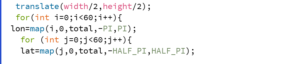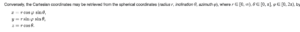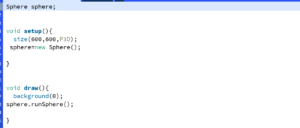# Generative Art Piece

#### Description& Inspiration:

`I wanted to create something simple yet eye catching, the first thing that came to mind was something like this, the picture on the  left  is a picture  of   mars,  while  the  right  is  how  I  imagined  I  can  bring  it  to  life  in  processing.`

#### Process:

`My focus in this project was creating an organized code and figuring out how to use Object-Oriented Programming rather than creating a complicated design. I started out as follows,  by laying out the needed standard functions such as setup() and draw(). Then I started planning and laying out all the variables I knew I was going to need. Starting out with drawing the actual sphere, the prices was quite long and a bit confusing however, after a lot of research and a lot of help from The Coding Train, I was able to get started. In order to create a sphere like shape (without resorting to the basic sphere function on processing),  I needed a longitude value (which is 'I' in the for loop) and latitude value (which is 'j' in the for loop).````with the above code only, the screen was still blank, in order to continue drawing the sphere the formulas for converting from latitude and logitude to coordinates were needed. Where theta refers to longitude and phi refers to latitude.
formulas are as follows :``````Then I needed to give motion to the sphere by using rotateX() and rotateY(), then I used point(x,y,z) to actually finish the drawing.

I also wanted to change the color of the sphere from red to yellow when any key is pressed so I used the mouse clicked for red and mouse pressed for yellow.

```

### Final Project:

`I created two versions: one where I have decreased the detail level of the sphere and how fine the resolution is and one where I increased it.`

This is the increased version:

This is the decreased version:

`I also really wanted to tidy up my code and make it easy to understand to I created two function, displaySphere() and colorChange(), and added them both two one big function which is runSphere(), so the final code only had sphere.runSphere() in it.`#### Difficulties:

```I would say that this is definitely the most challenging assignment I have gotten in the course so far in my opinion, the actual design was not very easy but what I found most difficult was trying to organize my code and figuring out the class. However, the way my code looks at the end was very worth it, and I will be able to use it later in other projects much easier.

Sources:
The Coding Train: Spherical Geometry video```
```Sphere sphere;

void setup(){
size(600,600,P3D);
sphere=new Sphere();

}

void draw(){
background(0);
sphere.runSphere();

}```
```class Sphere{

float r;
int total;
float x;
float y;
float z;
float lon;
float lat;

Sphere(){

r=200;
total=100;
x=r*sin(lon)*cos(lat);
y=r*sin(lon)*sin(lat);
}

void runSphere(){

displaySphere();
colorChange();
}

void displaySphere(){
background(0);
noFill();
lights();

//I needed a longitute value (which is 'i') and latitude value (which is 'j')
translate(width/2,height/2);
for(int i=0;i<150;i++){
lon=map(i,0,total,-PI,PI);
for (int j=0;j<150;j++){
lat=map(j,0,total,-HALF_PI,HALF_PI);

//as per the formulas for converting from latitude and logitude where theta refers to longitude and phi refers to latitude.
// formulas are as follows :
//x=rsin(theta)cos(phi)
//y=rsin(theta)sin(phi)
//z=r*cos(theta)
x=r*sin(lon)*cos(lat);
y=r*sin(lon)*sin(lat);
z=r*cos(lon);

//this if for the movement of the sphere (rotation) using mouse events
rotateX(mouseY);
rotateY(mouseX);

point(x,y,z);
}
}
}

//changing the color from yellow to red by using mouse events as well
void colorChange(){
if (mousePressed){
stroke(255,0,0);
} else if (keyPressed)
{
stroke(142,142,62);
}
}
}```